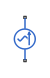# AC Current Source

Ideal sinusoidal current source

•Libraries:
Simscape / Foundation Library / Electrical / Electrical Sources

## Description

The AC Current Source block represents an ideal current source that maintains sinusoidal current through it, independent of the voltage across its terminals.

The output current is defined by the following equation:

`$I={I}_{0}\cdot \mathrm{sin}\left(2\pi \cdot f\cdot t+\phi \right)$`

where:

• I is current.

• I0 is peak amplitude.

• f is frequency.

• φ is phase shift.

• t is time.

The positive direction of the current flow is indicated by the arrow.

## Ports

### Conserving

expand all

Electrical conserving port associated with the source positive terminal.

Electrical conserving port associated with the source negative terminal.

## Parameters

expand all

Peak current amplitude.

Phase shift in angular units.

Current frequency, specified in Hz or units directly convertible to Hz (where Hz is defined as 1/s). For example, kHz and MHz are valid units, but rad/s is not.

## Version History

Introduced in R2007a